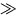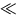# Force

In this section we introduce the concept of force. We discuss Newton's laws, which describe the way a body responds to a net force. We discuss frictional forces and the way they can be mathematically represented. We study several applications of Newton's laws.

Idea: Force is the cause of motion in classical mechanics. Classical mechanics deals with systems of size10- 10 m (atomic dimensions) and velocity3.0 x 108 m/s (the speed of light).

Note:

• Force is a vector.
• There are two kinds of forces:
• Contact Forces - involve physical contact between objects. Examples: the force involved in kicking a ball, pulling a wagon, compressing a spring, etc.
• Field forces - don't involve physical contact between objects. Examples: the gravitational force and the electromagnetic force.